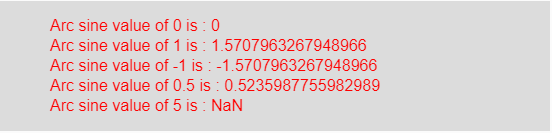# p5.js asin() function

The asin() function in p5.js is used to calculate the inverse of sine (arc sine). If the input value range is -1 to 1 then it returns the range between -π/2 to π/2.

Syntax:

`asin(value)`

Parameters: This function accepts a single parameter value which stores the domain of asin() function.

Return Value: It returns the arc sine of given value.

Below program illustrates the asin() function in p5.js:

Example: This example uses asin() function to get arc sine of a value.

 `function` `setup() {  ` `  `  `    ``// Create Canvas of given size ` `    ``createCanvas(550, 130);  ` `}  ` `  `  `function` `draw() {  ` `      `  `    ``// Set the background color  ` `    ``background(220);  ` `      `  `    ``// Initialize the parameter ` `    ``// with some values ` `    ``let a = 0;  ` `    ``let b = 1;  ` `    ``let c = -1; ` `    ``let d = 0.5; ` `    ``let e = 5; ` `      `  `    ``// Call to asin() function  ` `    ``let v = asin(a); ` `    ``let w = asin(b); ` `    ``let x = asin(c); ` `    ``let y = asin(d); ` `    ``let z = asin(e); ` `      `  `    ``// Set the size of text  ` `    ``textSize(16);  ` `      `  `    ``// Set the text color  ` `    ``fill(color(``'red'``));  ` `    `  `    ``// Getting arc sine value  ` `    ``text(``"Arc sine value of 0 is : "` `+ v, 50, 30); ` `    ``text(``"Arc sine value of 1 is : "` `+ w, 50, 50); ` `    ``text(``"Arc sine value of -1 is : "` `+ x, 50, 70); ` `    ``text(``"Arc sine value of 0.5 is : "` `+ y, 50, 90); ` `    ``text(``"Arc sine value of 5 is : "` `+ z, 50, 110);      ` `}  `

Output:Note: If the value is greater than 1 or less than -1 then it returns NaN.

Reference: https://p5js.org/reference/#/p5/asin

Whether you're preparing for your first job interview or aiming to upskill in this ever-evolving tech landscape, GeeksforGeeks Courses are your key to success. We provide top-quality content at affordable prices, all geared towards accelerating your growth in a time-bound manner. Join the millions we've already empowered, and we're here to do the same for you. Don't miss out - check it out now!

Previous
Next